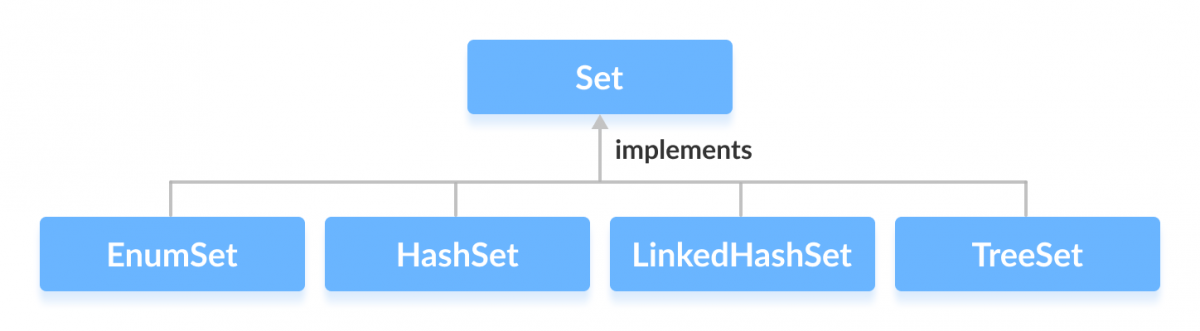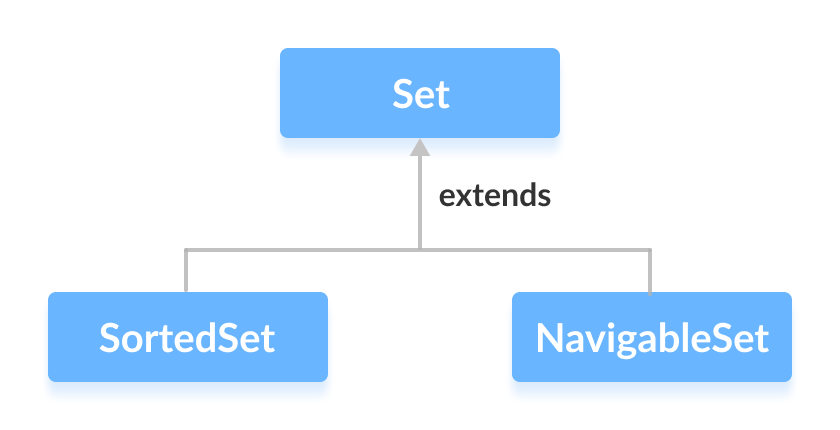# Java Set Interface

The `Set` interface of the Java `Collections` framework provides the features of the mathematical set in Java. It extends the `Collection` interface.

Unlike the `List` interface, sets cannot contain duplicate elements.

## Classes that implement Set

Since `Set` is an interface, we cannot create objects from it.

In order to use functionalities of the `Set` interface, we can use these classes:

These classes are defined in the `Collections` framework and implement the `Set` interface.## Interfaces that extend Set

The `Set` interface is also extended by these subinterfaces:## How to use Set?

In Java, we must import `java.util.Set` package in order to use `Set`.

``````// Set implementation using HashSet
Set<String> animals = new HashSet<>();
``````

Here, we have created a `Set` called animals. We have used the `HashSet` class to implement the `Set` interface.

## Methods of Set

The `Set` interface includes all the methods of the `Collection` interface. It's because `Collection` is a super interface of `Set`.

Some of the commonly used methods of the `Collection` interface that's also available in the `Set` interface are:

• addAll() - adds all the elements of the specified collection to the set
• iterator() - returns an iterator that can be used to access elements of the set sequentially
• remove() - removes the specified element from the set
• removeAll() - removes all the elements from the set that is present in another specified set
• retainAll() - retains all the elements in the set that are also present in another specified set
• clear() - removes all the elements from the set
• size() - returns the length (number of elements) of the set
• toArray() - returns an array containing all the elements of the set
• contains() - returns `true` if the set contains the specified element
• containsAll() - returns `true` if the set contains all the elements of the specified collection
• hashCode() - returns a hash code value (address of the element in the set)

To learn about more methods of the `Set` interface, visit Java Set (official Java documentation).

## Set Operations

The Java `Set` interface allows us to perform basic mathematical set operations like union, intersection, and subset.

• Union - to get the union of two sets x and y, we can use `x.addAll(y)`
• Intersection - to get the intersection of two sets x and y, we can use `x.retainAll(y)`
• Subset - to check if x is a subset of y, we can use `y.containsAll(x)`

## Implementation of the Set Interface

1. Implementing HashSet Class

``````import java.util.Set;
import java.util.HashSet;

class Main {

public static void main(String[] args) {
// Creating a set using the HashSet class
Set<Integer> set1 = new HashSet<>();

// Add elements to the set1
System.out.println("Set1: " + set1);

// Creating another set using the HashSet class
Set<Integer> set2 = new HashSet<>();

System.out.println("Set2: " + set2);

// Union of two sets
System.out.println("Union is: " + set2);
}
}
``````

Output

```Set1: [2, 3]
Set2: [1, 2]
Union is: [1, 2, 3]
```

To learn more about `HashSet`, visit Java HashSet.

2. Implementing TreeSet Class

``````import java.util.Set;
import java.util.TreeSet;
import java.util.Iterator;

class Main {

public static void main(String[] args) {
// Creating a set using the TreeSet class
Set<Integer> numbers = new TreeSet<>();

// Add elements to the set
System.out.println("Set using TreeSet: " + numbers);

// Access Elements using iterator()
System.out.print("Accessing elements using iterator(): ");
Iterator<Integer> iterate = numbers.iterator();
while(iterate.hasNext()) {
System.out.print(iterate.next());
System.out.print(", ");
}

}
}
``````

Output

```Set using TreeSet: [1, 2, 3]
Accessing elements using iterator(): 1, 2, 3,
```

To learn more about `TreeSet`, visit Java TreeSet.

Now that we know what `Set` is, we will see its implementations in classes like `EnumSet`, `HashSet`, `LinkedHashSet` and `TreeSet` in the next tutorials.